﻿ Board Paper of Class 12 2022 Chemistry Theory Term 1 Set4 Solutions | Aasoka

# Board Paper of Class 12 2022 Chemistry Theory Term 1 Set4 code-56/1/4 - Solutions

(This section consists of 25 multiple choice questions with over all choice to attempt any 20 questions. In case more than desirable number of questions are attempted, only first 20 questions will be considered for evaluation.).

## Which one of the following pairs will not form an ideal solution ?

1. Benzene and Toluene
2. n-Hexane and n-Heptane
3. Ethanol and Acetone
4. Bromoethane and Chloroethane

Ans. (c)

Ethanol and acetone do not form ideal solution because hydrogen bonding between ethanol molecules is weakened by acetone which leads to positive deviation from Raoult’s law.

## When NaCl is doped with , there will be a formation of :

1. Anion vacancies
2. Cation vacancies
3. Both cation and anion vacancies
4. F-centre

Ans. (b)

When molten NaCl is doped with Sr2+, some of the Sr2+ ions occupy sites of Na+ ions creating vacancies of Na+ ions as each Sr2+ will replace two Na+ ions.

## The structure of Oleum is: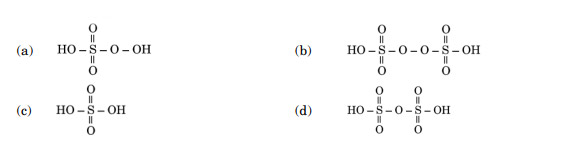Ans. (d)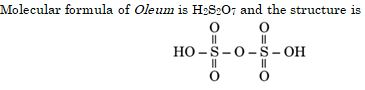## The C – O – C bond angle in the ether molecule is :

1. 111
2. 90
3. 120
4. 180

Ans. (a)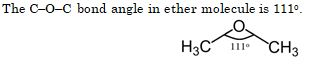## Which of the following reagents will not convert ethyl alcohol into ethyl chloride ?

1. ${\mathrm{PCl}}_{5}$
2. $\mathrm{NaCl}$
3. ${\mathrm{SOCl}}_{2}$
4. $\mathrm{HCl}/{\mathrm{ZnCl}}_{2}$

Ans. (b)

Hydroxyl group does not act as a good leaving group and does not displaced by a nucleophile. To facilitate the reaction, -OH group of alcohol is need to be protonated by an acid such as sulphuric acid.

## Nucleotides are composed of a :

1. pentose sugar and phosphoric acid
2. nitrogenous base and phosphoric acid
3. nitrogenous base and a pentose sugar
4. nitrogenous base, a pentose sugar and phosphoric acid

Ans. (d)

Nucleotide forms DNA strand and each nucleotide is made by:

1. Nitrogenous base
2. Pentose sugar
3. Pentose sugar

## The oxidation state of − 3 is least stable in :

1. N
2. Bi
3. As
4. P

Ans. (b)

Stability of -3 oxidation state decreases down the group due to increase in size and metallic character.

## Amorphous solids may also be classified as

1. supercooled solids
2. superheated liquids
3. supercooled liquids
4. superheated solids

Ans. (c)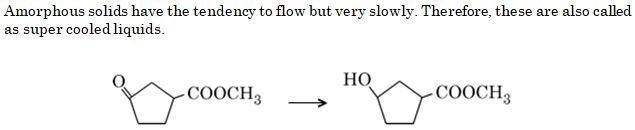## Which of the following reagents should be used to carry out the above conversion?

1. LiAlH4
2. NaBH4
3. Zn-Hg/HCl
4. KMnO4

Ans. (b)

NaBH4 is a weak reducing agent than LiAlH4. It reduces only ketone group in the given compound.

## An azeotropic solution of two liquids has a boiling point higher than either of the two when it :

1. shows a negative deviation from Raoult’s law
2. shows a positive deviation from Raoult’s law
3. is saturated
4. shows no deviation from Raoult’s law

Ans. (a)

In case of negative deviation from Raoult’s law, new bonds formed between molecules are stronger than the intermolecular forces between respective constituents. The tendency of molecules to escape decrease and boiling point increases.

## Which of the following crystals will show metal deficiency defect ?

1. $\mathrm{NaCl}$
2. $\mathrm{ZnO}$
3. $\mathrm{FeO}$
4. $\mathrm{AgCl}$

Ans. (c)

In the crystal of FeO, some Fe2+ ions are missing and for the loss of every three Fe2+ ions, two Fe3+ ions present causing metal deficiency.

## Phenol on being heated with concentrated ${{H}}_{2}{\mathrm{SO}}_{4}$ and then with concentrated gives :

1. o-nitrophenol
2. 2,4,6-trinitrophenol
3. p-nitrophenol
4. m-nitrophenol

Ans. (b)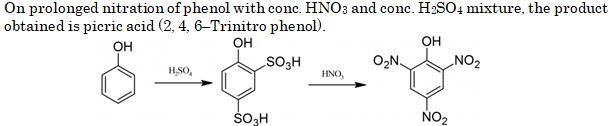## ${\mathrm{O}}_{3}$ reacts with KI solution to produce :

1. ${\mathrm{KIO}}_{3}$

Ans. (d)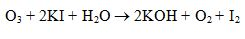## α-D-Glucose and β-D-Glucose differ from each other with respect to the :

1. Number of – OH groups
2. Configuration at the C-1 carbon
3. Size of the hemiacetal ring
4. Configuration at the C-5 carbon

Ans. (b)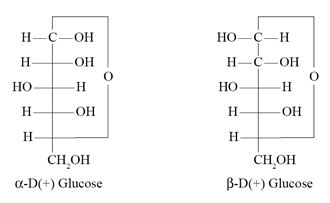## Lucas reagent produces cloudiness immediately with :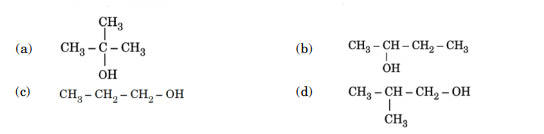Ans. (a)

Tertiary alcohols give turbidity immediately with Lucas reagent as they form halides easily.

## Which of the following is most reactive towards nucleophilic substitution reaction?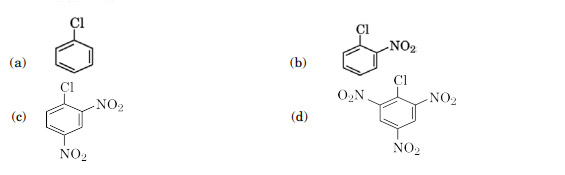Ans. (d)

In case of haloarenes, presence of an electron withdrawing group (such as -NO2) at ortho- and para- position increases their reactivity.

## Pressure does not have any significant effect on solubility of solids in liquids because :

1. Solids are highly compressible
2. Liquids are highly compressible
3. Solubility of solid in liquid is directly proportional to partial pressure
4. Solids and liquids are highly incompressible

Ans. (d)

Solids and liquids are highly incompressible due to absence of intermolecular spaces.

## Main product in the following reaction is: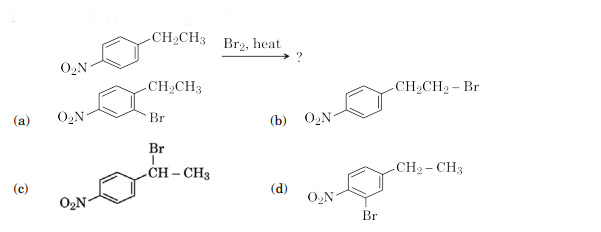Ans. (c)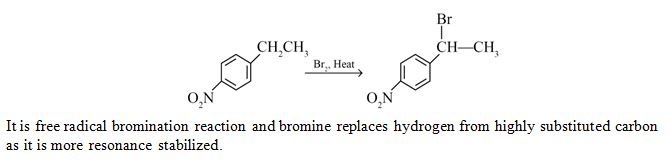## Which of the following forms strong p$\pi$ − p$\pi$ bonding ?

1. ${\mathrm{P}}_{4}$
2. ${\mathrm{N}}_{2}$
3. ${\mathrm{Sb}}_{4}$
4. ${\mathrm{As}}_{4}$

Ans. (b)

Only nitrogen forms p$\mathrm{\pi }$—p$\mathrm{\pi }$ multiple bonds due to small size and high electronegativity. Other elements of group-15 do not form p$\mathrm{\pi }$—p$\mathrm{\pi }$ multiple bonds due to large and highly diffused atomic orbitals.

## Which of the following halogens can replace all other halogens from their halidecompounds ?

1. ${\mathrm{F}}_{2}$
2. ${\mathrm{Cl}}_{2}$
3. ${\mathrm{Br}}_{2}$
4. ${\mathrm{I}}_{2}$

Ans.(a)

Fluorine (F) can replace all other halogens from their halide compound because of small size and high electronegativity.

## The functional unit that is repeated in a protein molecule is :

Ans. (c)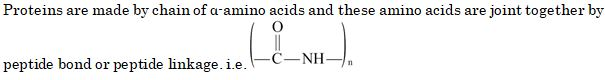## The lowest boiling point of ‘He’ is due to :

1. Its inertness
2. Its high polarizability
3. Its small size
4. Weak dispersion forces between its atoms

Ans. (d)

In case of helium, only weak dispersion forces are present leading to low boiling point.

## Major products formed by heating (CH3)3C – O – CH2 – CH3 with HI are :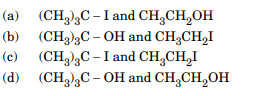Ans. (a)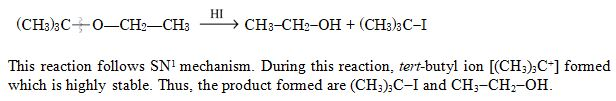## The osmotic pressure of a solution increases if :

1. The volume of the solution is increased
2. The number of solute molecules is increased
3. Temperature is decreased
4. Solution constant (R) is increased

Ans. (b)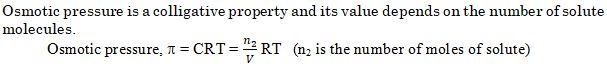## Chlorine reacts with hot and concentrated NaOH to give: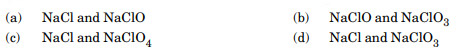Ans. (d)

(This section consists of 24 multiple choice questions with overall choice to attempt any 20 questions. In case more than desirable number of questions are attempted, only first20 questions will be considered for evaluation).

## Vapour pressure of dilute aqueous solution of glucose is 750 mm Hg at 373 K. The mole fraction of solute is :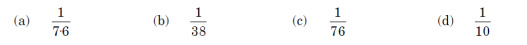Ans. (c)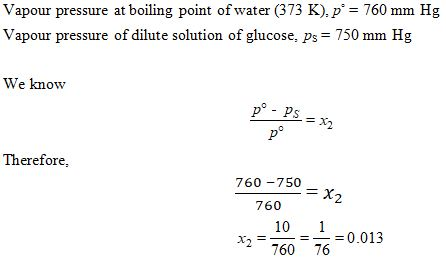## The bases that are common in both DNA and RNA are :

4. Guanine, Uracil and Thymine

Ans. (a)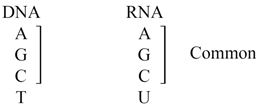## A compound (X) with the molecular formula C3H8O can be oxidised to another compound (Y) whose molecular formula is C3H6O2. The compound (X) may be: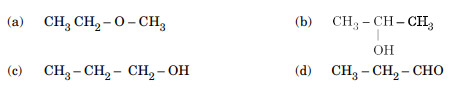Ans. (c)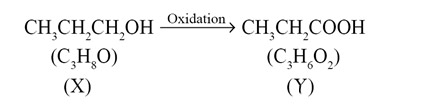## Which reagent is required for one step conversion of benzene diazonium chloride to bromobenzene ?

1. ${\mathrm{PBr}}_{3}$
2. $\mathrm{HBr}$
3. ${\mathrm{Cu}}_{2}{\mathrm{Br}}_{2}$
4. ${\mathrm{Br}}_{2}$

Ans. (c)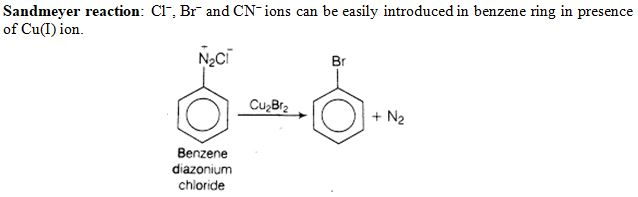## The number of lone pair of electrons on $\mathrm{Xe}$ in , ${\mathrm{XeF}}_{4}$ and ${\mathrm{XeF}}_{6}$ compounds are respectively :

1. 4, 3 and 2
2. 2, 3 and 1
3. 3, 2 and 0
4. 3, 2 and 1

Ans. (d)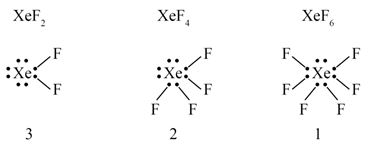## Which form of sulphur shows paramagnetic behaviour ?

1. ${\mathrm{S}}_{8}$
2. ${\mathrm{S}}_{4}$
3. ${\mathrm{S}}_{2}$
4. ${\mathrm{S}}_{6}$

Ans.(c)

In vapour state of sulphur partly exists as S2 molecule and S2 molecule has two unpaired electrons in the anti-bonding ${\mathbit{\pi }}^{\mathbf{*}}$ orbitals.

## An element with density 3 g cm-3 forms a bcc lattice with edge length of 3 × 10-8 cm. The molar mass of the element is: (NA= 6 × 1023 mol-1)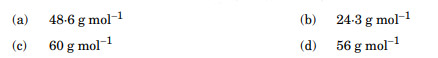Ans. (b)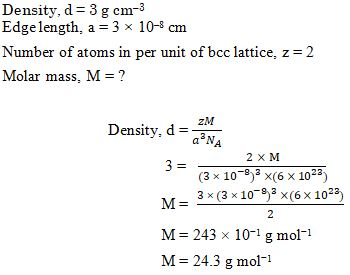## In the following reaction: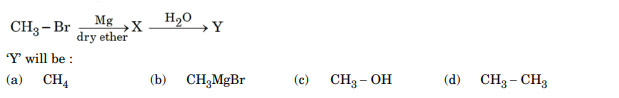Ans. (a)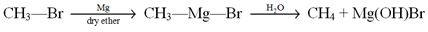## Which of the following has the greatest reducing power ?

1. HI
2. HBr
3. HCl
4. HF

Ans. (a)

HI is the strongest reducing agent among halogen acids because of lowest bond dissociation energy.

## The freezing point of a 0.2 molal solution of a non-electrolyte in water is: (Kf for water = 1.86 K kg mol-1)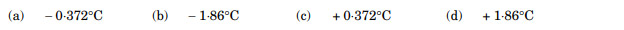Ans. (a)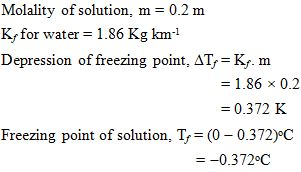## In a bcc structure, the packing efficiency is approximately :

1. 58%
2. 68%
3. 32%
4. 74%

Ans. (b)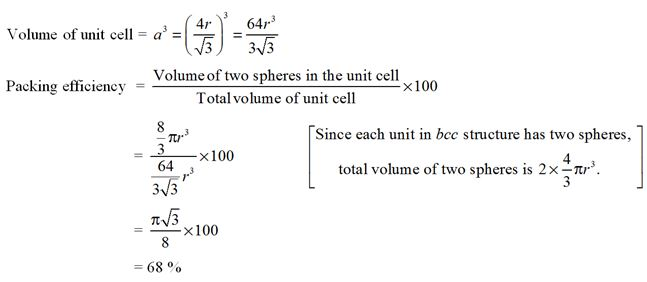## ${\mathrm{NO}}_{2}$ gas dimerises because :

1. It is acidic in nature
2. It contains even number of valence electrons
3. It contains odd number of valence electrons
4. It is inert at room temperature

Ans. (c)

NO2 contains odd number of valance electrons. On dimerisation, it gives N2O4 molecule having even number of electrons.

## A compound forms hcp structure. The number of tetrahedral voids in 0.5 mol of it is: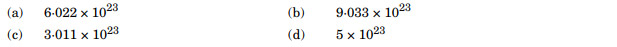Ans. (a)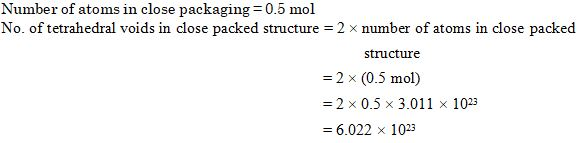## XeF2 on reaction with PF5 forms: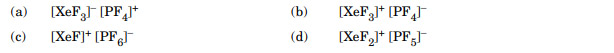Ans. (c)

PF5 act as a fluoride ion acceptor.

## Arrange the following compounds in decreasing order of their acidic character :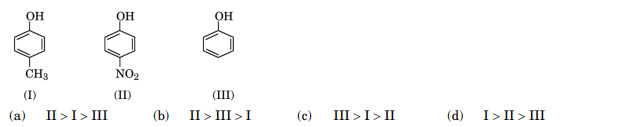Ans. (b)

-NO2 is an electron withdrawing group and stabilises the phenoxide ion while -CH3 is an electron donating group and do not favours the phenoxide ion formation.

## Which of the following compounds undergoes racemisation on hydrolysis with aqueous KOH?Ans. (d)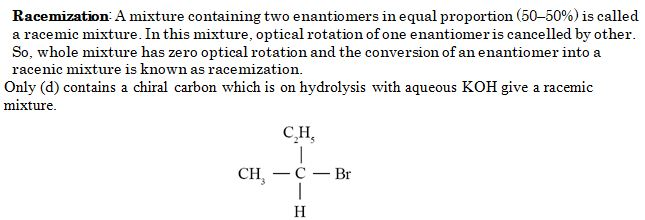## Which of the following is not true ?

1. Fluorine exhibits only – 1 oxidation state.
2. Among halide ions, is the strongest oxidising agent.
3. F − F bond has lower bond dissociation enthalpy than Cl − Cl bond.
4. Fluorine forms only one oxoacid

Ans. (b)

Among halide ions, iodine is the weakest oxidising agent due to its large size.

## The IUPAC name of isobutyl bromide is :

1. 1-bromo-3-methylbutane
2. 3-bromo-2-methylpropane
3. 2-bromo-2-methylpropane
4. 1-bromo-2-methylpropane

Ans. (d)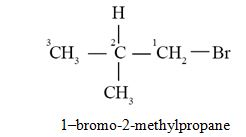## Chlorobenzene when treated with sodium in dry ether gives Diphenyl. It is called :

1. Wurtz reaction
2. Fittig reaction
3. Wurtz-Fittig reaction
4. Friedel-Crafts reaction

Ans. (b)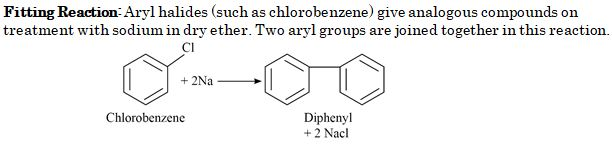Question Nos. 45 to 49 are Assertion (A) and Reason (R) type questions. Given below are two statements labelled as Assertion (A) and Reason (R). Select the most appropriate answer from the options given below
1. Both Assertion (A) and Reason (R) are true and Reason (R) is the correct explanation of Assertion (A).
2. Both Assertion (A) and Reason (R) are true, but Reason (R) is not the correct explanation of Assertion (A).
3. Assertion (A) is true, but Reason (R) is false.
4. Assertion (A) is false, but Reason (R) is true.

## Assertion (A) : ${\mathrm{NH}}_{3}$ is less basic than ${\mathrm{PH}}_{3}$Reason (R) : Nitrogen is more electronegative than phosphorus.

Ans. (d)

Ammonia (NH3) is more basic than PH3 as the size of nitrogen is smaller and electron density is more than phosphorous.

## Assertion (A) : Osmotic pressure is a colligative property. Reason (R) : Osmotic pressure of a solution depends on the molar concentration of solute at any temperature T

Ans. (a)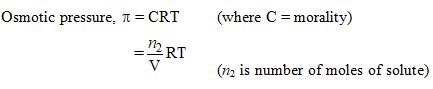## Assertion (A) : Aryl halides are extremely less reactive towards nucleophilic substitution reaction. Reason (R) : Halogen atom shows +I effect in Aryl halides.

Ans. (c)

Aryl halides are extremely less reactive towards nucleophilic substitution reaction due to resonance effect.

## Assertion (A) : Due to Frenkel defect there is no effect on density of solid. Reason (R) : Ions shift from its normal site to an interstitial site in Frenkel defect.

Ans. (a)

Frenkel defect is also called as dislocation effect. The smaller ion (cation) is dislocated to an interstitial site and no change in density observed.

## Assertion (A) : Ozone is a powerful oxidising agent in comparison to O2. Reason (R) : Ozone is thermodynamically stable with respect to oxygen.

Ans. (c)

Ozone is a powerful oxidising agent as it liberates nascent oxygen easily. Ozone is thermodynamically unstable with respect to ozone and it produces heat by decomposing to oxygen

(This section consists of 6 multiple choice questions with an overall choice to attempt any 5 questions. In case more than the desirable number of questions are attempted, only the first 5 questions will be considered for evaluation).

## Match the following: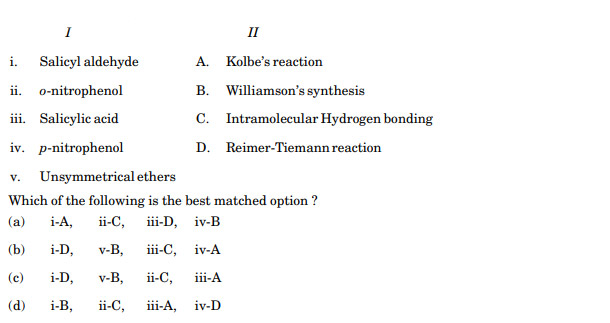Ans. (c)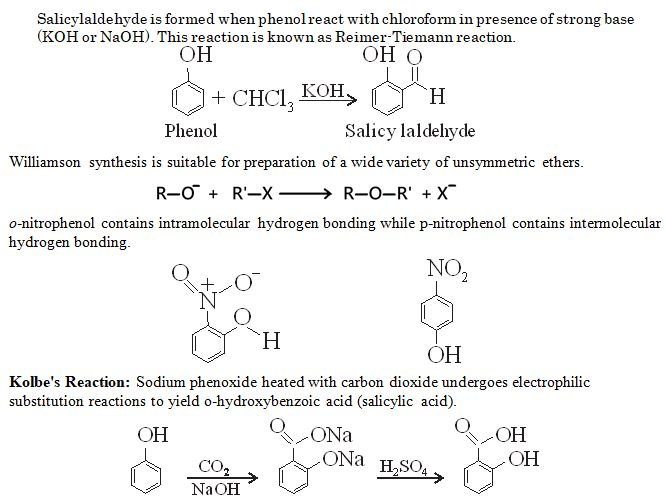## Which of the following analogies is correct :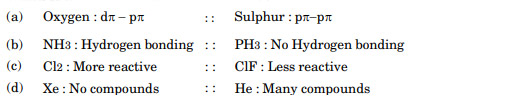Ans. (b)

Oxygen contains no d-orbital for bonding.

Cl2 is less reactive than ClF.

Xe form many compounds but He not.

## Complete the following analogy: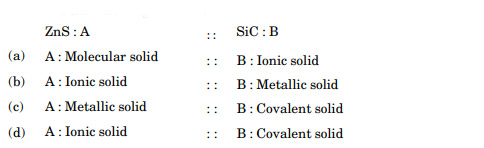Ans. (d)

ZnS - Ionic solid (contains ionic bonds as zinc is metal and sulphur is a non-metal) SiC - Covalent solid (contains covalent bonds as both elements are non-metals)

###### Case – Study

Read the passage given below and answer the following question nos. 53 – 55.

Carbohydrates are polyhydroxy aldehydes or ketones and are also called saccharides. Glucose is an example of monosaccharides. Glucose (C6H12O6) is an aldohexose and its open chain structure was assigned on the basis of many reactions as evidences like presence of carbonyl group, presence of straight chain, presence of five -OH groups, etc. Glucose is correctly named as D-(+)-Glucose. Glucose is found to exist in two different crystalline forms which are named as $\mathrm{\alpha }$ and $\mathrm{\alpha }$. Despite having the aldehyde group, glucose does not give 2, 4-DNP test.

## Which of the following represents D-(+)-Glucose ?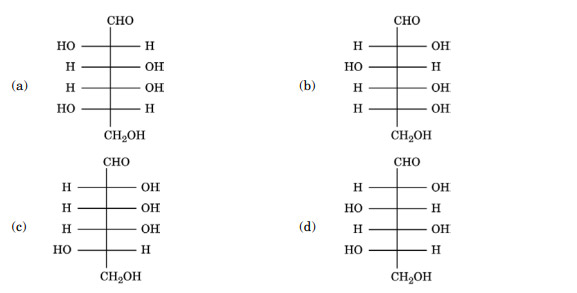Ans. (b)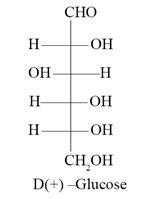## Glucose on oxidation with ${\mathrm{HNO}}_{3}$ gives a dicarboxylic acid called saccharic acid. This result validates the fact that Glucose possesses :

1. − CHO group
2. − OH group
3. Data is not made available to everybody.
4. both − CHO and − groups at the terminals of the chain

Ans. (d)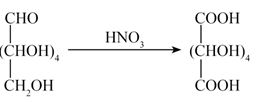## The pentaacetate of glucose does not react with − indicating the absence of :

1. − OH group
2. − CHO group
3. − COOH group
4. group

Ans. (b)

Carbonyl group (>C=O) reacts with hydroxylamine (NH2OH) to form an oxime. No reaction with hydroxylamine confirms absence of carbonyl group in pentacetate of glucose.